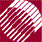ECE532 Biomedical Optics © 1998 Steven L. Jacques, Scott A. Prahl Oregon Graduate Institute

# Time-resolved Monte Carlo

## Variables used in program

The variables and arrays used by the program are declared here with brief explanations. We shall more fully discuss each parameter as it is used within the program.

```/* Propagation parameters */
double	x, y, z;    /* photon position */
double	ux, uy, uz; /* photon trajectory as cosines */
double  uxx, uyy, uzz;	/* temporary values used during SPIN */
double	s;          /* step sizes. s = -log(RND)/mus [cm] */
double	costheta;   /* cos(theta) */
double  sintheta;   /* sin(theta) */
double	cospsi;     /* cos(psi) */
double  sinpsi;     /* sin(psi) */
double	psi;        /* azimuthal angle */
double	i_photon;   /* current photon */
short   photon_status;  /* flag = ALIVE=1 or DEAD=0 */

/* time and pathlength variables */
short	NTpts;      /* number of timepoints */
double  T;       /* timepoints at which concentration is mapped */
double  LT;      /* total pathlengths at timepoints */
double  Lmax;       /* maximum timepoint and pathlength considered */
double  L;          /* total photon pathlength */
short	it;         /* index to timepoint */

/* other variables */
double  c;          /* speed of light in medium */
double	Csph;  /* photon concentration C[ir=0..100][it=0-3] */
double	F;  /* fluence rate */
double	mus;        /* scattering coefficient [cm^-1] */
double	g;          /* anisotropy [-] */
double	nt;         /* tissue index of refraction */
double	Nphotons;   /* number of photons in simulation */
short	NR;         /* number of radial positions */
double	r;          /* radial position */
double  dr;         /* radial bin size */
short	ir;         /* index to position */
double  shellvolume;  /* volume of shell at radial position r */

/* dummy variables */
double  rnd;            /* assigned random value 0-1 */
double  s1, x1, y1, z1; /* temporary values */
short	i, j;           /* dummy indices */
double	u, temp, sum;   /* dummy variables */
FILE*	target;         /* pointer to output file */
```

NextMC |